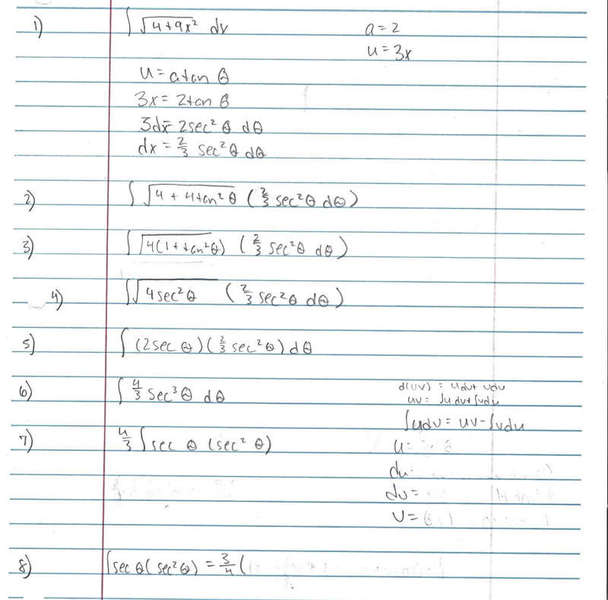# Applying Integration by Parts & Trig Substitution

## Homework Statement

$$\int\sqrt{4+9x^{2}}dx$$

## Homework Equations

Pythagorean Identities?

## The Attempt at a Solution

I find it sort of cumbersome to use the special formatting here, so I hope it is okay that I just photocopied my work on paper.

You can see how far I made it, but now I am stuck. I am pretty sure I need to use integration by parts to finish the problem, but I am not sure how.Sorry about all the erasure marks, they showed up kind of dark.

rock.freak667
Homework Helper
So all you need to do is have

u=secθ and dv=sec2θ dθ

and you know that ∫ u dv = uv - ∫ v du.

So all you need to do is have

u=secθ and dv=sec2θ dθ

and you know that ∫ u dv = uv - ∫ v du.

How do I integrate dv? I know the integral of sec θ = ln ( sec θ + tan θ) + c

Matterwave
Gold Member
The integral of sec^2(x) is tan(x)+C. You can figure this out by simply noting that the derivative of tan=sin/cos is sec^2.

The integral of sec^2(x) is tan(x)+C. You can figure this out by simply noting that the derivative of tan=sin/cos is sec^2.

Wow, I can't believe I missed this! Thank you!

Alright, I still seem to have a problem. I now have
$$(3/4)(secθtanθ)-\int tan^{2}θsecθ$$

Where do I go from here?

Last edited:
Is there anyone that can help me finish this problem?

rock.freak667
Homework Helper
Is there anyone that can help me finish this problem?

you'll need to put back in 1+tan2θ = sec2θ (yes this will give you back an integral of sec3θ)

Now remember you got something like

∫sec3θ dθ = secθ tanθ - ∫tan2θsecθ dθ

you'll see that you can put the ∫sec3θ dθ (that you get from the substitution) back on the left side. If you don't get what I mean, write it all out and you should hopefully see it.

I am still not seeing it.

Currently, I have
$$\int sec^{3}Θ = (3/4)(secΘtanΘ - \int tan^{2}ΘsecΘ)$$

rock.freak667
Homework Helper
I am still not seeing it.

Currently, I have
$$\int sec^{3}Θ = (3/4)(secΘtanΘ - \int tan^{2}ΘsecΘ)$$

So when you put in $tan^2 \theta = sec^2\theta -1$

$$\int sec^3 \theta d\theta = \frac{3}{4}sec\theta tan\theta - \int sec\theta (sec^2\theta-1) d\theta$$

$$\int sec^3 \theta d\theta = \frac{3}{4}sec\theta tan\theta - \int sec^3 \theta d\theta -\int sec \theta d\theta$$

So you can move that ∫ sec3θ dθ to the left side to get 2∫ sec3θ dθ.

Okay, thats what I thought you wanted me to do. I am now left with this:

$$(7/4)\int sec^{3}Θ = (3/4)secΘtanΘ - (3/4)ln\left|secΘ + tanΘ\right| + C$$

Now I need to manipulate the fraction so as to get
$$(4/3)\int sec^{3}Θ$$

How do I do this?

rock.freak667
Homework Helper
Okay, thats what I thought you wanted me to do. I am now left with this:

$$(7/4)\int sec^{3}Θ = (3/4)secΘtanΘ - (3/4)ln\left|secΘ + tanΘ\right| + C$$

Now I need to manipulate the fraction so as to get
$$(4/3)\int sec^{3}Θ$$

How do I do this?

multiply both sides by 4/7 and then multiply by 4/3. The left side would give ∫ (4/3)sec3θ dθ.

Okay, I got it now. Thanks for all your help!